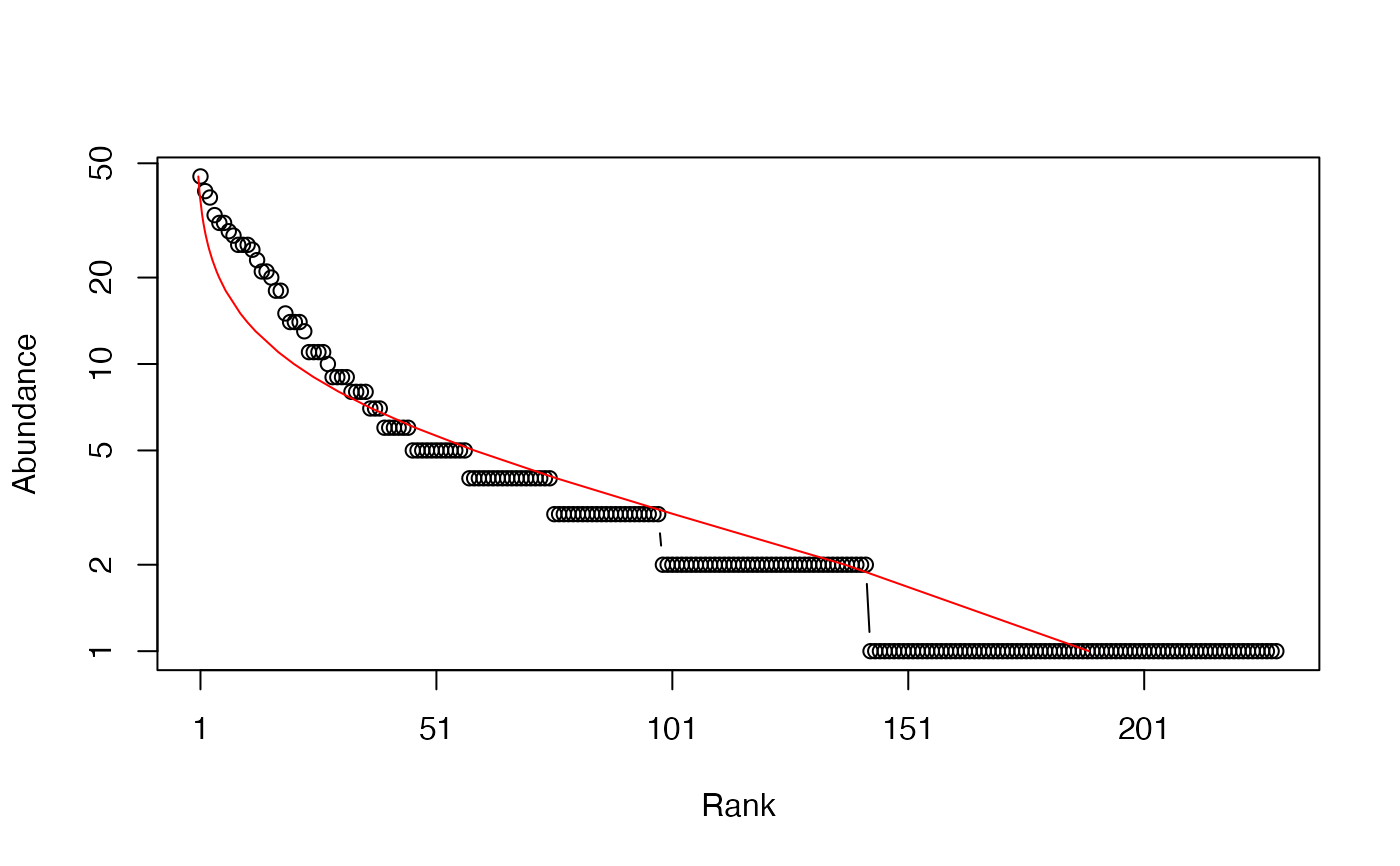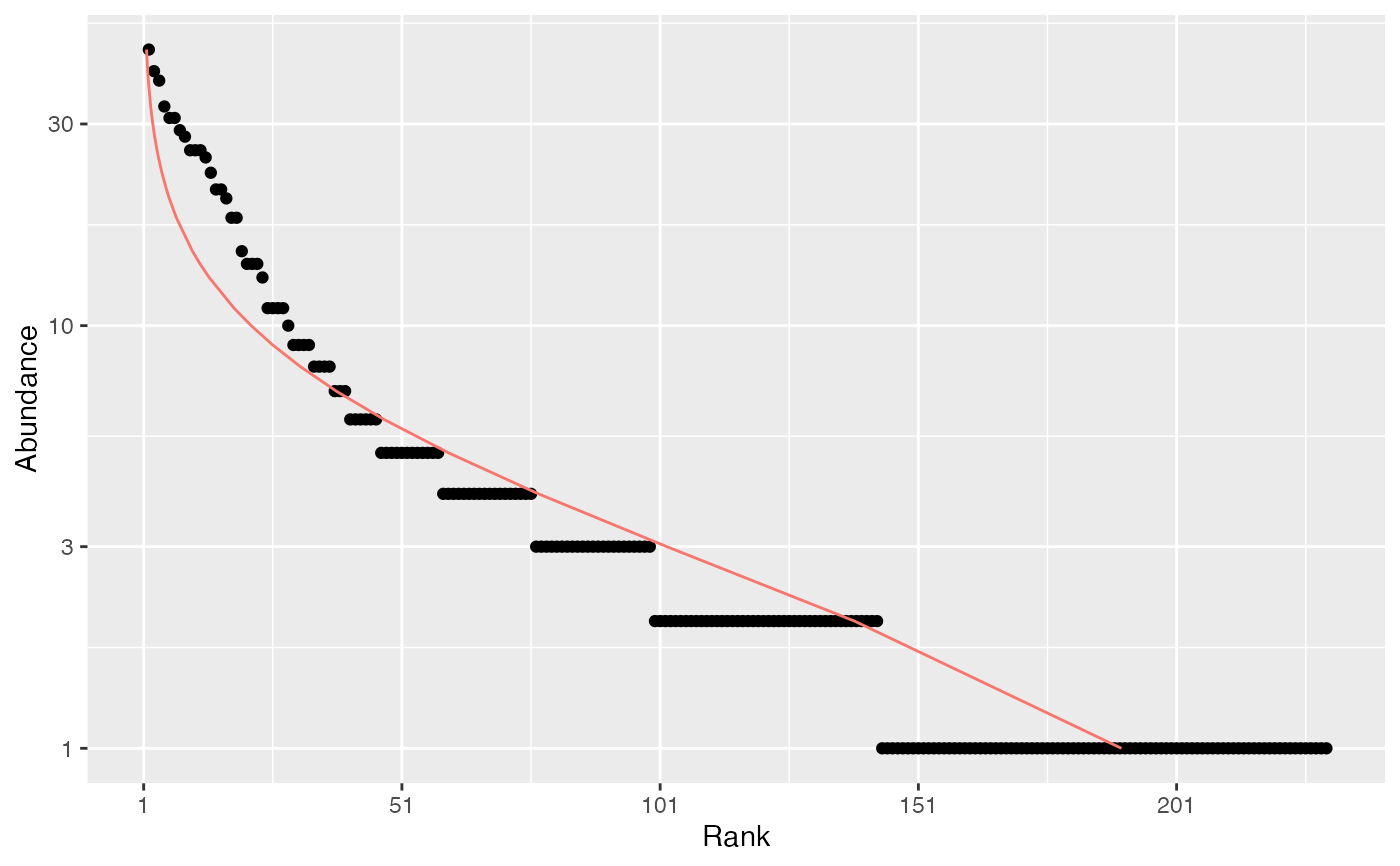A Species Distribution is a (preferably named) vector containing species abundances or probabilities.

as.SpeciesDistribution(x, ...)
# S3 method for data.frame
as.SpeciesDistribution(x, ...)
# S3 method for integer
as.SpeciesDistribution(x, ...)
# S3 method for numeric
as.SpeciesDistribution(x, ...)
# S3 method for SpeciesDistribution
autoplot(object, ..., Distribution = NULL,
ylog = TRUE, main = NULL, xlab = "Rank", ylab = NULL,
pch = ggplot2::GeomPoint$default_aes$shape,
col = ggplot2::GeomPoint$default_aes$colour,
cex = ggplot2::GeomPoint$default_aes$size)
# S3 method for SpeciesDistribution
plot(x, ..., Distribution = NULL,
type = "b", log = "y", main = NULL, xlab = "Rank", ylab = NULL)
is.SpeciesDistribution(x)
as.ProbaVector(x, ...)
# S3 method for data.frame
as.ProbaVector(x, ...)
# S3 method for integer
as.ProbaVector(x, Correction = "None", Unveiling = "None",
RCorrection = "Jackknife", JackOver = FALSE, JackMax = 10,
CEstimator = "ZhangHuang", q = 0, ..., CheckArguments = TRUE)
# S3 method for numeric
as.ProbaVector(x, Correction = "None", Unveiling = "None",
RCorrection = "Jackknife", JackOver = FALSE, JackMax = 10,
CEstimator = "ZhangHuang", q = 0, ..., CheckArguments = TRUE)
is.ProbaVector(x)
as.AbdVector(x, ...)
# S3 method for data.frame
as.AbdVector(x, Round = TRUE, ...)
# S3 method for integer
as.AbdVector(x, ...)
# S3 method for numeric
as.AbdVector(x, Round = TRUE, ...)
is.AbdVector(x)

## Arguments

x

An object.

object

An object.

Distribution

The distribution to fit on the plot. May be "lnorm" (log-normal), "lseries" (log-series), "geom" (geometric) or "bstick" (broken stick). If NULL, no distribution is fitted. See rCommunity for the description of these distributions.

Round

If TRUE (by default), values of x are set to integer to create an AbdVector. This is useful if original abundances are not integers (this is often the case for MetaCommunity abundances which are the product of probabilities by the number of individuals) and integer values are required (for example to calculate the bootstrap confidence interval of a community profile).

Correction

A string containing one of the possible corrections to estimate a probability distribution: "None" (no correction, the default value), or "Chao2013", "Chao2015", "ChaoShen" to estimate the probability of the observed species in the asymptotic distribution.

Unveiling

A string containing one of the possible unveiling methods to estimate the probabilities of the unobserved species: "None" (default, no species is added), "unif" (uniform: all unobserved species have the same probability) or "geom" (geometric: the unobserved species distribution is geometric).

RCorrection

A string containing a correction recognized by Richness to evaluate the total number of species. "Jackknife" is the default value. An alternative is "Rarefy" to estimate the number of species such that the entropy of order q of the asymptotic distribution rarefied to the observed sample size equals the actual entropy of the data.

JackOver

If TRUE, retain the jackknife order immediately superior to the optimal one, usually resulting in the overestimation of the number of species. Default is FALSE. Ignored if RCorrection is not "Jackknife".

JackMax

The highest jackknife order allowed. Default is 10. Allowed values are between 1 and 9.

CEstimator

A string containing an estimator recognized by Coverage to evaluate the sample coverage. "ZhangHuang" is the default value.

q

A number: the order of entropy. Default is 0 for richness. Used only to estimate asymptotic probability distributions with RCorrection equal to "Rarefy". Then, the number of unobserved species is fitted so that the entropy of order q of the asymptotic probability distribution at the observed sample size equals the actual entropy of the data.

type

The plot type, see plot.

log

The axis to plot in log scale, e.g. "xy" for both axes. Default is "y".

main

The main title of the plot. if NULL (by default), there is no title.

xlab

The X axis label, "Rank" by default.

ylab

The Y axis label. if NULL (by default), "Probability" or "Abundance" is chosen according to the object class.

ylog

Logical; if TRUE (by default), the Y-axis of the plot is log scaled.

pch

The plotting characters. See points.

col

The color of the geom objects. See "Color Specification" in par.

cex

The character expansion (size) of the points. See points.

...

Additional arguments to be passed to plot. Unused elsewhere.

CheckArguments

Logical; if TRUE, the function arguments are verified. Should be set to FALSE to save time when the arguments have been checked elsewhere.

## Details

SpeciesDistribution objects include AbdVector and ProbaVector objects.

as.AbdVector just sets the class of the numeric or integer x so that appropriate versions of community functions (generic methods such as Diversity) are applied. Abundance values are rounded (by default) to the nearest integer.

as.ProbaVector normalizes the vector so that it sums to 1. If Correction is not "None", the observed abundance distribution is used to estimate the actual species distribution. The list of species will be changed: zero-abundance species will be cleared, and some unobserved species will be added. First, observed species probabilities are estimated folllowing Chao and Shen (2003), i.e. input probabilities are multiplied by the sample coverage, or according to more sophisticated models: Chao et al. (2013, single-parameter model), or Chao et al. (2015, two-parameter model). The total probability of observed species equals the sample coverage. Then, the distribution of unobserved species can be unveiled: their number is estimated according to RCorrection (if the Jackknife estimator is chosen, the JackOver argument allows using the order immediately over the optimal one). The coverage deficit (1 minus the sample coverage) is shared by the unobserved species equally (Unveiling = "unif", Chao et al., 2013) or according to a geometric distribution (Unveiling = "geom", Chao et al., 2015).

These functions can be applied to data frames to calculate the joint diversity (Gregorius, 2010).

SpeciesDistribution objects can be plotted. The plot method returns the estimated parameters of the fitted distribution. The broken stick has no parameter, so the maximum abundance is returned.

## Note

Fisher's alpha (Fisher et al., 1943) is estimated to fit the log-series distribution. The estimation is done by the fisher.alpha function of package vegan. It may differ substantially from the estimation returned by optimal.theta from package untb.

## Author

Eric Marcon <Eric.Marcon@agroparistech.fr>, Bruno Herault <Bruno.Herault@cirad.fr>

rgeom, rlnorm, rCommunity, RAClnorm

## Examples

# Load Paracou data (number of trees per species in two 1-ha plot of a tropical forest)
data(Paracou618)
# Ns is the total number of trees per species
Ns <- as.AbdVector(Paracou618.MC$Ns) # Whittaker plot, poorly fitted by a log-normal distribution plot(Ns, Distribution = "lnorm")#>$mu
#>  0.9519812
#>
#> \$sigma
#>  1.011383
#>
# ggplot version
autoplot(Ns, Distribution = "lnorm")